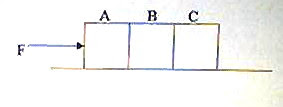## Wednesday, July 14, 2010

### EAMCET 2010 Questions (MCQ) on Surface Tension

Today we will discuss two questions on surface tension which appeared in EAMCET 2010 question papers.

The following multiple choice question appeared in EAMCET 2010 (Engineering) question paper:

The excess pressure inside a spherical soap bubble of radius 1 cm is balance by a column of oil (Sp. gravity 0.8) 2 mm high. The surface tension of oil is

(1) 3.92 N/m

(2) 0.0392 N/m

(3) 0. 392 N/m

(4) 0.00392 N/m

The excess pressure ∆P insie a spherical bubble of radius r is given by

∆P = 4 T/r where T is the surface tension.

The pressure p exerted by a liquid column of height h is given by

p = hρg where ρ is the density of the liquid.

Therefore we have

4 T/r = hρg from which T = rhρg/4

Substituting for known values, T = (1×10–2×2×10–3×0.8×103×10)/4 = 0.004 N/m [Option (4)].

[If you substitute g = 9.8 ms–2 instead of 10 ms–2 (as we did above for convenience), you will get the answer as 0.00392 N/m].

Here is the question which appeared in EAMCET 2010 (Agriculture and Medicine) question paper:

A spherical liquid drop of diameter D breaks up to n identical spherical drops. If the surface tension of the drop is ‘σ’, the change in energy in this process is

(1) πσD2(n1/3 – 1)

(2) πσD2(n2/3 – 1)

(3) πσD2(n – 1)

(4) πσD2(n4/3 – 1)

The surface energy (E1) of a drop of radius R is given by

E1 = 4πR2σ

Or E1 = πD2σ where D is the diameter of the drop.

When a drop of radius R breaks into n identical droplets, the radius r of each droplet is given by (on equating the volumes)

(4/3) πR3 = n×(4/3) πr3

Therefore, r = R/n1/3

The total surface energy (E2) of all the n droplets is given by

E2 = n×4πr2σ = n×4π (R/n1/3) 2σ = 4πR2σn1/3

Or, E2 = πD2σn1/3

The change in energy is E2 E1 = πσD2(n1/3 – 1)

## Saturday, July 10, 2010

### Multiple Choice Questions Involving Friction

Any intelligent fool can make things bigger and more complex…

It takes a touch of genius and a lot of courage to move in the opposite direction.

Albert Einstein

Here are two questions (MCQ) on friction, which may easily tempt you to answer incorrectly:(1) Three identical wooden blocks A, B and C, each of mass 1 kg, are placed on a rough horizontal surface as shown. A horizontal force F acts on the block A and the system of masses moves with an acceleration of 2 ms–2. If the coefficient of friction between the blocks and the horizontal surface is 0.3 and the gravitational acceleration is 10 ms–2, the force exerted by block A on block B is

(a) 2 N

(b) 4 N

(c) 6 N

(d) 8N

(e) 10 N

The block A pushes the blocks B and C and produces an acceleration in them. But when the blocks A and B move, an opposing frictional force Ff is produced. The opposing frictional force is given by

Ff = μmg where μ is the coefficient of friction, m is the total mass of blocks A and B and g is the gravitational acceleration.

Therefore, Ff = 0.3×2×10 = 6 N.

The resultant force acting on block B is FB Ff where FB is the force exerted by block A on block B. Therefore, we have

FB Ff = ma = 2×2 since the resultant force moves blocks A and B with an acceleration of 2 ms–2.

Therefore, FB – 6 = 4 so that FB = 10 N.(2) A block of mass M slides down with uniform velocity from the top A to the bottom B of a rough inclined plane of angle θ and height H. If the coefficient of kinetic friction between the block and the plane is μk, the work done against friction during the motion of the block is

(a) MgH sin θ

(b) μkMgH sin θ

(c) μkMgH/ sin θ

(d) μkMgH

(e) MgH

When the block reaches the bottom of the incline, the gravitational potential energy of the block decreases by MgH. If the plane were smooth, the kinetic energy of the block would have increased by an equal amount. Since the kinetic energy of the block remains unchanged, it follows that the loss in the gravitational potential energy is equal to the work done against friction [Option (e)].

Now see two posts in this section here.